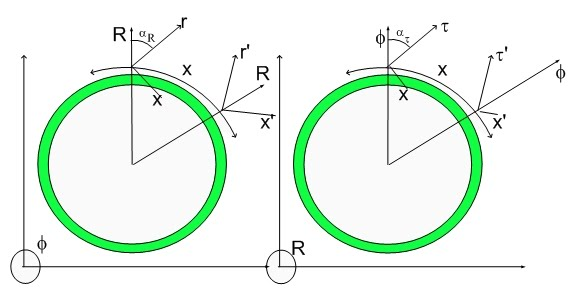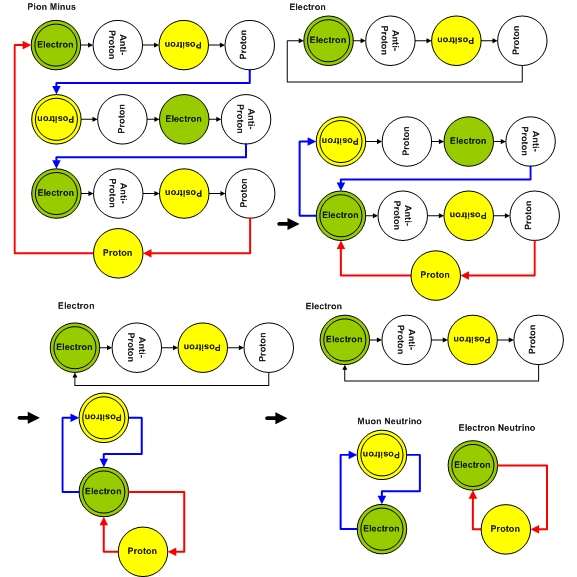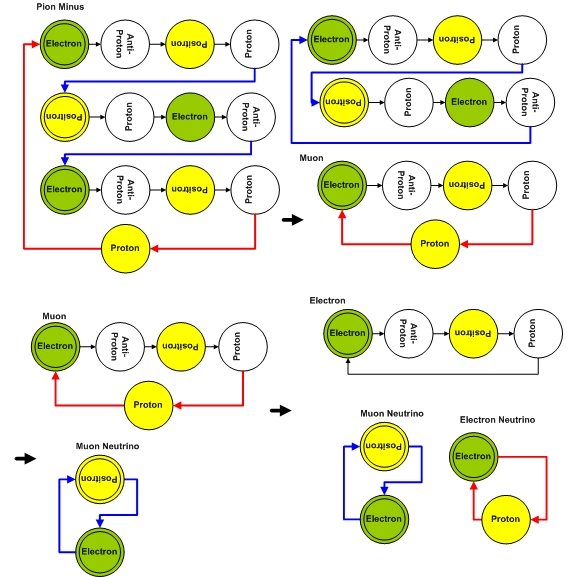# Pion Minus DecayPion Minus Decay

The Pion Minus is the simplest member of the Hyperon Family. It decays into two channels:
PionMinus=> Electron + NeutrinoElectron + NeutrinoMuon:The Electron-Positron connected with two blue transmutation notes is the NeutrinoMuon while the Electron-Proton states connected with two red transmutations notes is the NeutrinoElectron. The collapse of the Electron or Positron coherence into a single state is because the fundamental coherence does not introduce any strain into the fabric of space, thus those coherences are similar in "energy" or 3D Volume. Those two states have similar energies. One can easily envision decaying reactions where multiple electron-positron pairs are introduced and the neutrino nature is not changed, that is, its frequency is not synchronous with the fundamental dilator and thus it does not interact with the surroundings. An elecron and a positron dilator sequences of opposing spin have zero displacement volume. The transmutations notes is all that remains after their anihilation. This is a reduction into an irreducible representation of the original particle and releases little energy.

From these drawings one can answer the perennial question of the mass of a neutrino...:) It has inertial mass... but it doesn't have gravitational mass...:)

If you didn't read my Gallileo Gallilei Biggest Blunder, here is the retrospective review. Inertial mass is the 3D footprint of the dilator. There is obviously some 3D footprint, thus there is obviously inertial mass. The Gravitational mass has to do with the intensity AND FREQUENCY of the dilaton waves. The intensity has to do with the 4D displacement volume of the fundamental dilator within a cycle. Obviously, there is a 4D displacement volume. There is a FREQUENCY MISMATCH...which averages out all dilaton-dilator interaction resulting in a NULL Gravitational Mass..

The other channel is the following:
PionMinus=> MuonMinus + ElectronNeutrinowhere the Muon Minus decayed into an electron and a electron neutrino.

This decaying reaction was short-circuited in my previous blog. I write these blogs very quickly and managed not to correctly write the decay reaction. What I wrote was just the Pion Minus and Neutrino assignments.

Now, I finally have the chance correct that error. Please notice that I am considering that current experimentalists did not realize that two neutrinos are released as opposed to just one along the first channel, that is, what people call a Muon Neutrino contains two neutrinos - the Muon Neutrino per si and an Electron Neutrino, released in subsequent decays. In this assignment both a Muon Neutrino and an Electron Neutrino are released by the Pion Minus decay.

Current understanding is that only the Muon Neutrino is released..>:) My view is that the initial transitory state decays into the Muon and Electron Neutrino channel.

Cheers,

MP
Currently unrated

### New Comment

required

required (not published)

optional

required